### Home > A2C > Chapter 11 > Lesson 11.2.3 > Problem11-70

11-70.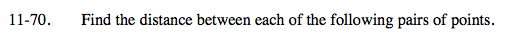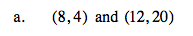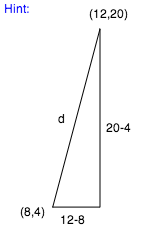$\sqrt{(20-4)^2+(12-8)^2}$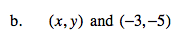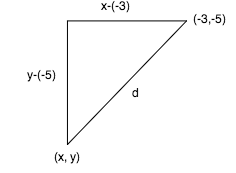$\sqrt{(y-(-5))^2+(x-(-3))^2}$